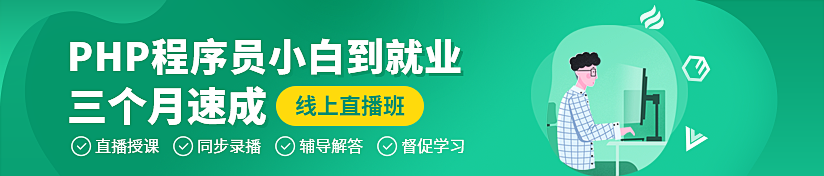# go语言常量有多少种

go语言常量有5种：布尔型常量、整数型常量、浮点型常量、复数常量和字符串型常量。Go语言中的常量使用关键字const定义，用于存储不会改变的数据，常量是在编译时被创建的，即使定义在函数内部也是如此，并且只能是布尔型、数字型（整数型、浮点型和复数）和字符串型。Go语言中的常量使用关键字 const 定义，用于存储不会改变的数据，常量是在编译时被创建的，即使定义在函数内部也是如此，并且只能是布尔型、数字型（整数型、浮点型和复数）和字符串型。由于编译时的限制，定义常量的表达式必须为能被编译器求值的常量表达式。

```const x = true
fmt.Print(x) //输出true```

```const x = 20
fmt.Print(x) //输出20```

```cOnstx= 0.618
fmt.Print(x) //输出%f0.618```

```const x = 'a'
fmt.Print(x) //输出97

const x = "a"
fmt.Print(x) //输出a```

```const x = 3 + 2i
fmt.Print(x) //输出%v(3+2i)```

Go语言的常量有个不同寻常之处。虽然一个常量可以有任意一个确定的基础类型，例如 int 或 float64，或者是类似 time.Duration 这样的基础类型，但是许多常量并没有一个明确的基础类型。

【示例 1】math.Pi 无类型的浮点数常量，可以直接用于任意需要浮点数或复数的地方：

```var x float32 = math.Pi
var y float64 = math.Pi
var z complex128 = math.Pi```

```const Pi64 float64 = math.Pi
var x float32 = float32(Pi64)
var y float64 = Pi64
var z complex128 = complex128(Pi64)```

【相关推荐：Go视频教程编程教学#### 相关文章推荐

• golang怎么检测变量是否为字符串• Go语言中类型与接口有什么关系• go语言的init()方法有什么用• go语言的beego是什么• go语言怎么进行强制类型转换
1/1